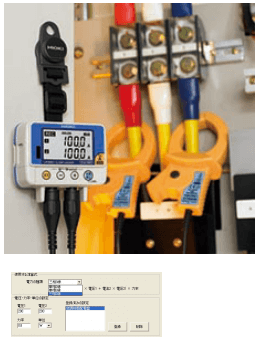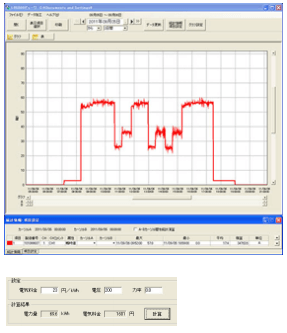# Measure Energy Savings Using a Small Clamp Logger

## Easily record the load current of equipment and understand your energy saving situation using the LR5051 Clamp Logger. Identify unnecessary loads and achieve load leveling.

■Highlights

- With one LR5051, the load current can be recorded on 2 channels by connecting an optional clamp sensor.
- The average, maximum, and minimum values during the recording interval can be recorded for approx. 2 days at a recording interval of 15 seconds and approx. 10 days at a recording interval of 1 minute.
- When recording only instantaneous values during the recording interval, recording can be performed for approx. 10 days at a recording interval of 15 seconds and approx. 40 days at a recording interval of 1 minute.
- The data can be displayed in graph format and electric power can be calculated easily using an LR5091 Communication Adapter and software included with the LR5092-20 Data Collector.

## Simplified electric power calculation function:

The electric power value for single-phase two-wire, single-phase three-wire, and three-phase three-wire systems can be easily calculated by just entering the voltage and power factor values.## Simplified electric power rate calculation function:

The electric power rate for a single-phase two-wire system can be calculated easily by just entering the voltage and power factor values.## (Note) Simplified electric power calculation and simplified electric power rate calculation:

The voltage and power factor are actually not measured so the calculation results are simplified. The calculated value does not agree with the actual electric power value in a circuit where the voltage and power factor change. Use a Clamp On Power HiTester to measure the electric power accurately.